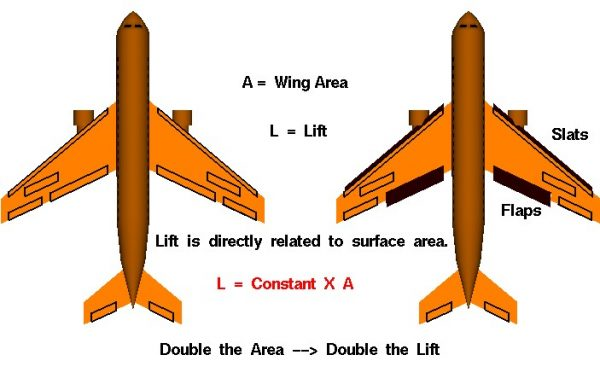# Effect of Size on Lift## Lift

The amount of lift generated by an object depends on the size of the object. Lift is an aerodynamic force and therefore depends on the pressure variation of the air around the body as it moves through the air. The total aerodynamic force is equal to the pressure times the surface area around the body. Lift is the component of this force perpendicular to the flight direction. Like the other aerodynamic force, drag, the lift is directly proportional to the area of the object. Doubling the area doubles the lift.

## Lift Equation and Coefficient

There are several different areas from which to choose when developing the reference area used in the lift equation. Since most of the lift is generated by the wings, and lift is the force perpendicular to the flight direction, the logical choice is the wing planform area. The planform area is the area of the wing as viewed from above the wing, looking along the “lift” direction. It is a flat plane, and is NOT the total surface area (top and bottom) of the entire wing, although it is almost half that number for most wings. We could, in theory, use the total surface area as the reference area. The total surface area is proportional to the wing planform area. Since the lift coefficient is determined experimentally, by measuring the lift and measuring the area and performing the necessary math to produce the coefficient, we are free to use any area which can be easily measured. If we choose the total surface area, the computed coefficient has a different value than if we choose the wing planform area, but the lift is the same, and the coefficients are related by the ratio of the areas.

## Surface Area

This slide shows the projected surface area for two different aircraft. The airplane on the left is shown in a cruise condition while the airplane on the right is shown in a takeoff or landing condition. Takeoff and landing are times of relatively low velocity, so to keep the lift high (to avoid the ground!) designers try to increase the wing area. This is done by sliding the flaps backwards along metal tracks and shifting the slats forward to increase the wing area. The next time you fly in an airliner, watch the wings during takeoff and landing to see the change in wing area.

Since aircraft come in many shapes and sizes, an aerodynamicist has to be able to compute the wing area for many different shapes. Most of these skills are learned in high school.

Provide feedback